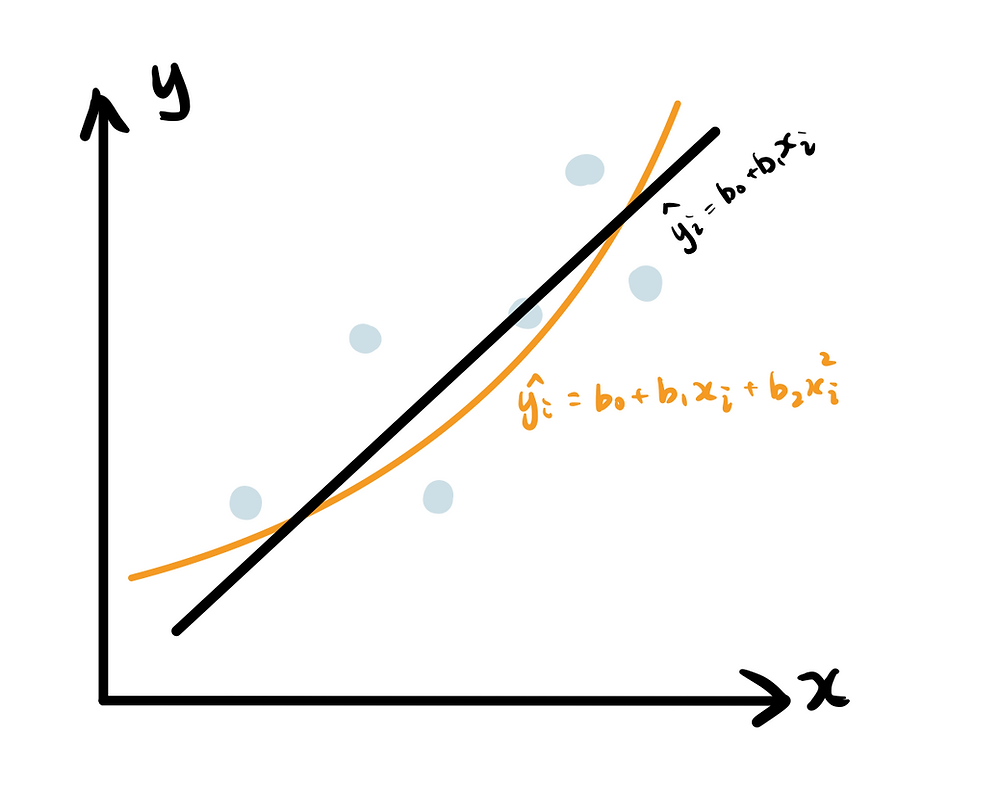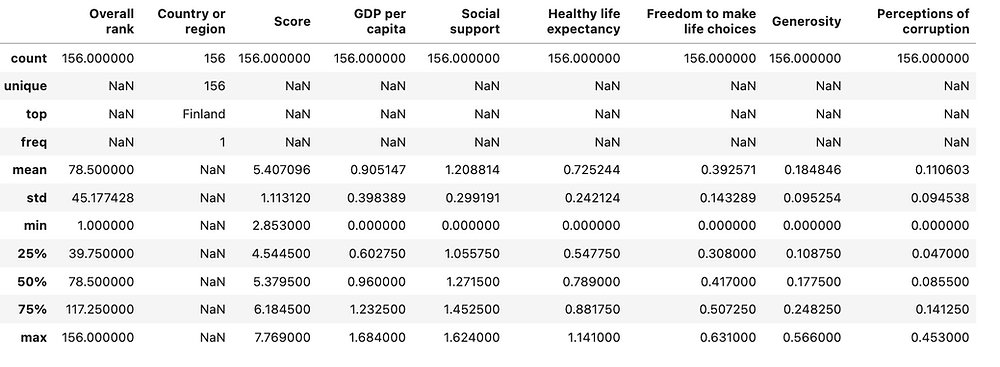Search

# Top Machine Learning Algorithms for Regression

Updated: Apr 18

## A Comprehensive Guide to Implementation and Comparison###### upgrade and grab the cheatsheet from our infographics gallery

In my previous post "Top Machine Learning Algorithms for Classification", we walked through common classification algorithms. Now let’s dive into the other category of supervised learning - regression where the output variable is continuous and numeric. There are four common types of regression models.

• Linear Regression

• Lasso Regression

• Ridge Regression

• Polynomial Regression

For people who prefer video walkthrough

## Linear Regression

Linear regression finds the optimal linear relationship between independent variables and dependent variables, thus makes prediction accordingly. The simplest form is y = b0 + b1x. When there is only one input feature, linear regression model fits the line in a 2 dimensional space, in order to minimize the residuals between predicted values and actual values. The common cost function to measure the magnitude of residuals is residual sum of squared (RSS).As more features are introduced, simple linear regression evolves into multiple linear regression y = b0 + b1x1 + b2x2 + ... + bnxn. Feel free to visit my article if you want the specific guide to simple linear regression model.

## Lasso RegressionLasso regression is a variation of linear regression with L1 regularization. Sounds daunting? Simply put, it adds an extra element to the residuals that regression models are trying to minimize. It is called L1 regularization because this added regularization term is proportional to the absolute value of coefficients - degree of 1. Compared to Ridge Regression, it is better at bringing the coefficients of some features to 0, hence a suitable technique for feature elimination. You’ll see in the later section “Feature Importance”.

## Ridge RegressionRidge regression is another regression variation with L2 regularization. So not hard to infer that the regularization term is based on the squared value of coefficients - degree of 2. Compared to Lasso Regression, Ridge Regression has the advantage of faster convergence and less computation cost.The regularization strength of Lasso and Ridge is determined by lambda value. Larger lambda values shrink down the coefficients values which makes the model more flattened and with less variance. Therefore, regularization techniques are commonly used for prevent model overfitting.

## Polynomial RegressionPolynomial regression is a variation of linear regression with polynomial feature transformation. It adds interaction between independent variable. PolynomialFeatures(degree = 2) is applied to transform input features to a maximum degree of 2. For example, if the original input features are x1, x2, x3, this expands features into x1, x2, x3, x1^2, x1x2, x1x3, x2^2, x2x3, x3^2. As the result, the relationship is no longer linear, instead provide a non-linear fit to the data.

## Regression Models in Practice

Let's implement and compare these 4 types of regression models, and explore how different lambda values affect model performance.

Please check out code snippet if you are interested in getting the full code of this project.

### 1. Objectives and Dataset Overview

This project aims to use regression models to make prediction of the country happiness scores based on other factors “GDP per capita”, “Social support”, Healthy life expectancy”, “Freedom to make life choices”, “Generosity” and “Perceptions of corruption”.

I used “World Happiness Report” dataset on Kaggle, which includes 156 entries and 9 features. df.describe() is applied to provide an overview of the dataset.### 2. Data Exploration and Feature Engineering

1) drop redundant features

Feature "Overall rank" is dropped as it is a direct reflection of the target “Score”. Additionally, “Country or Region” is dropped because it doesn’t bring any values to the prediction.

2) univariate analysis

Apply histogram to understand the distribution of each features. As shown below, “Social support” appears to be heavily left skewed whereas “Generosity” and “Perceptions of corruption” are right skewed - which informs the feature engineering tech